# Selina Solutions Concise Mathematics Class 6 Chapter 17: Idea of Speed, Distance and Time Exercise 17(A)

Selina Solutions Concise Mathematics Class 6 Chapter 17 Idea of Speed, Distance and Time Exercise 17(A) help students get a clear idea of topics and the steps to be followed in solving problems with ease. The solutions prepared in an understandable language, by expert faculty at BYJU’S, help students to achieve high marks in the final examinations. Shortcut tips and formulas are covered in a stepwise manner, for a better understanding of the concepts. Selina Solutions Concise Mathematics Class 6 Chapter 17 Idea of Speed, Distance and Time Exercise 17(A) PDF, can be downloaded from the available links below.

## Selina Solutions Concise Mathematics Class 6 Chapter 17: Idea of Speed, Distance and Time Exercise 17(A) Download PDF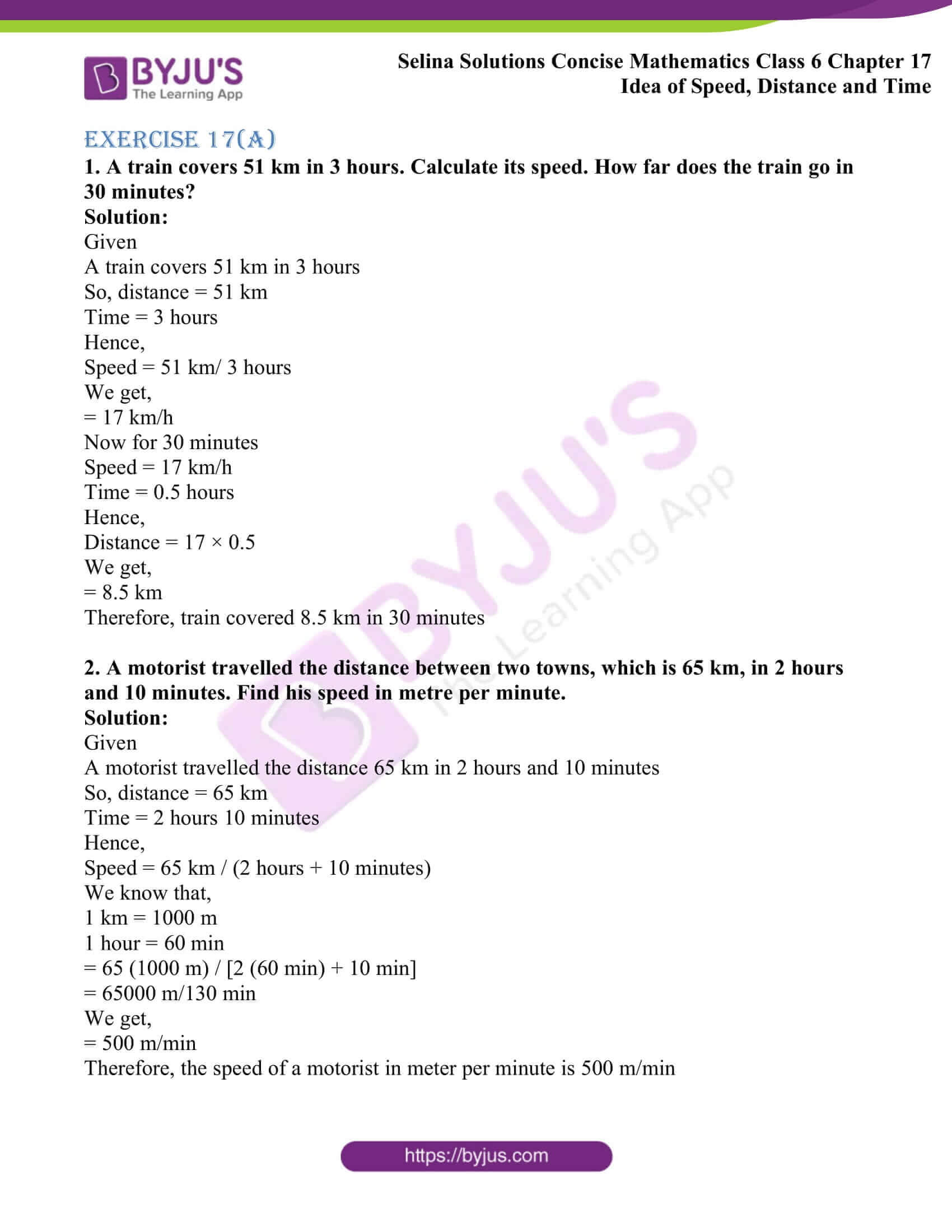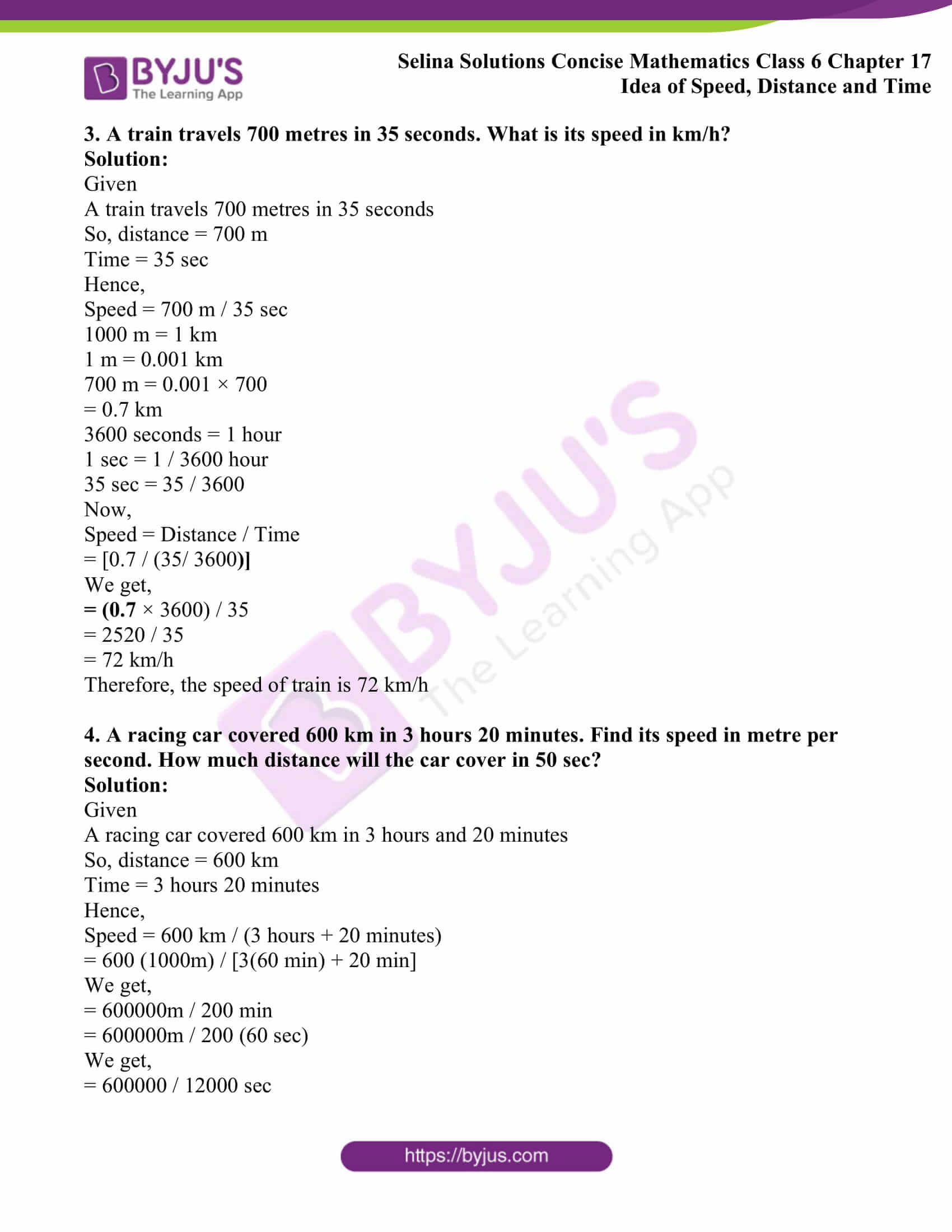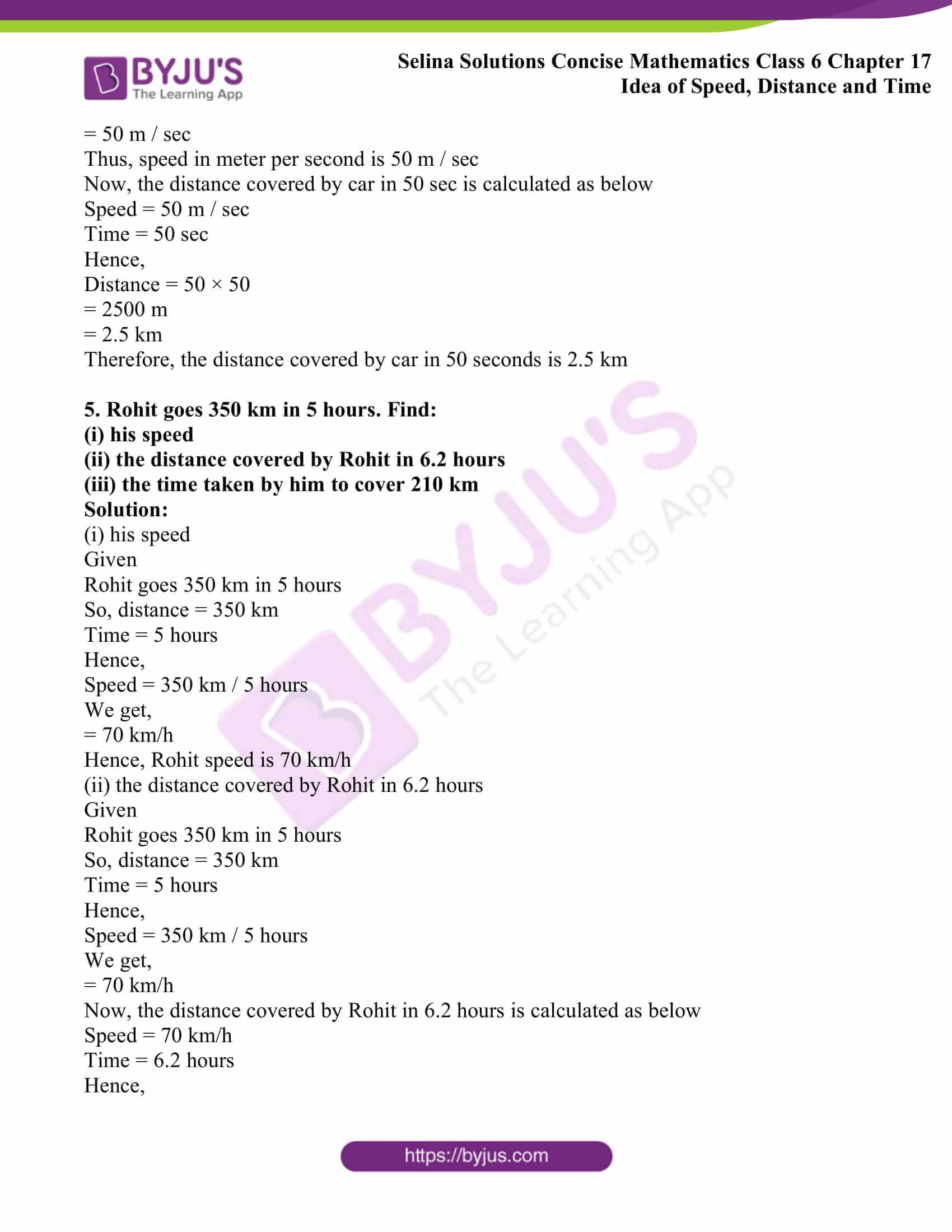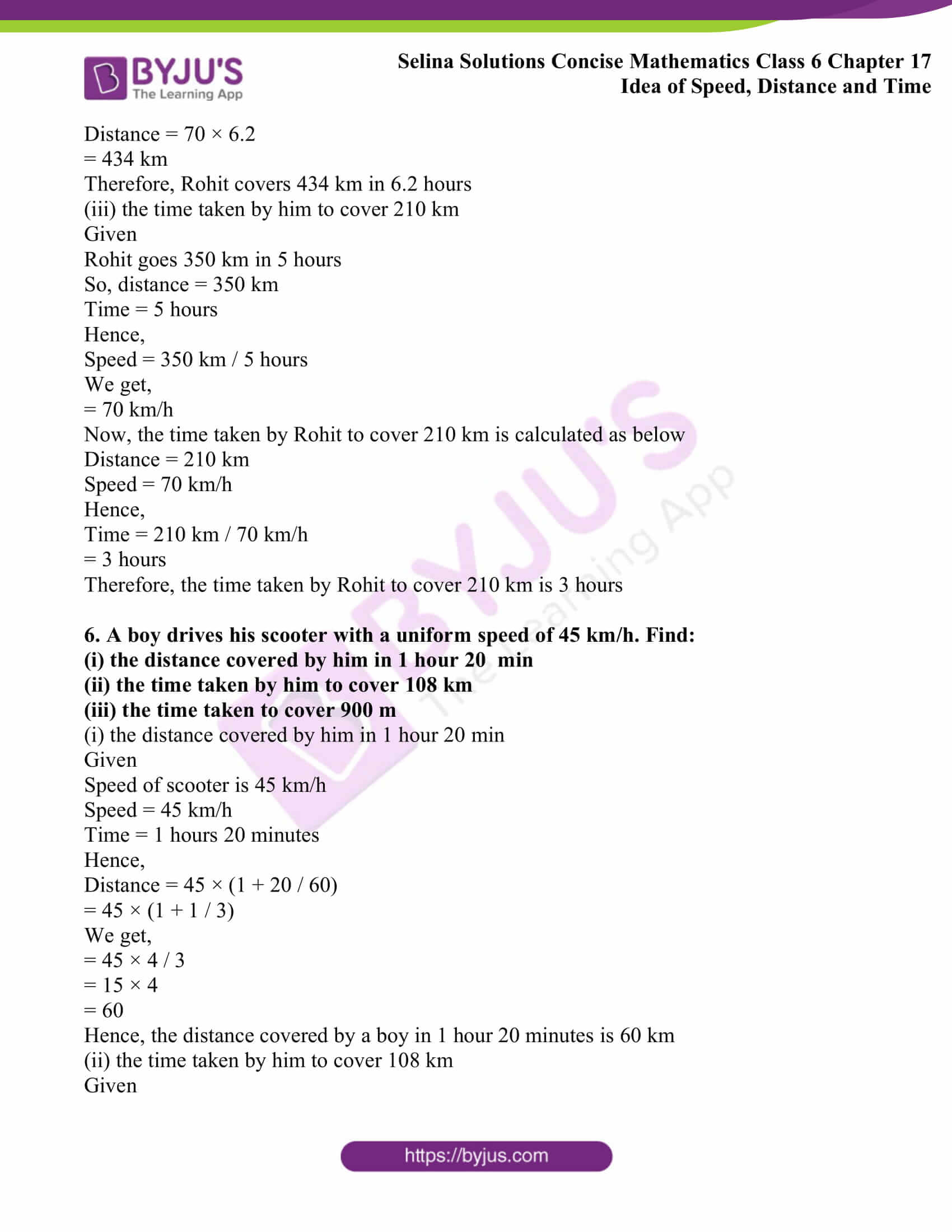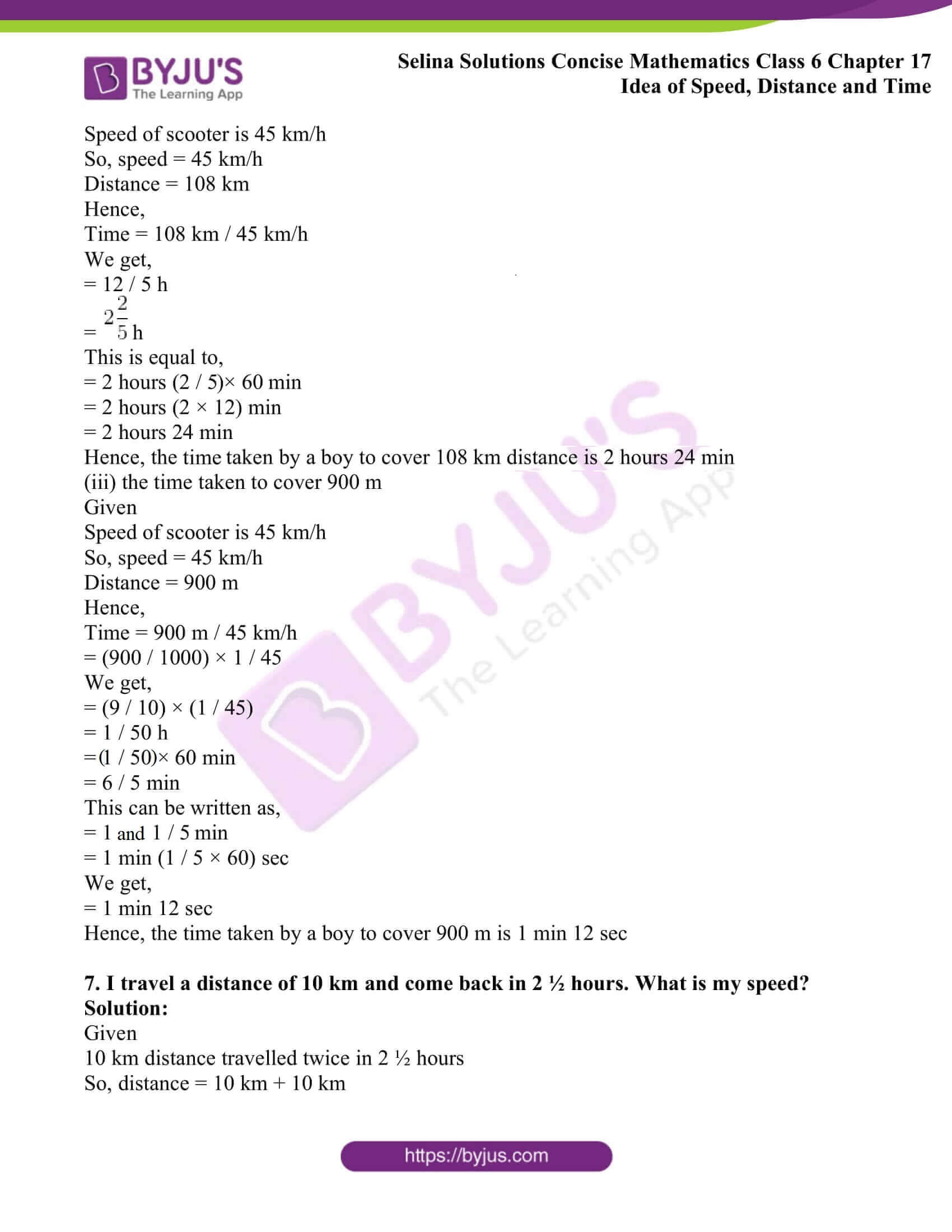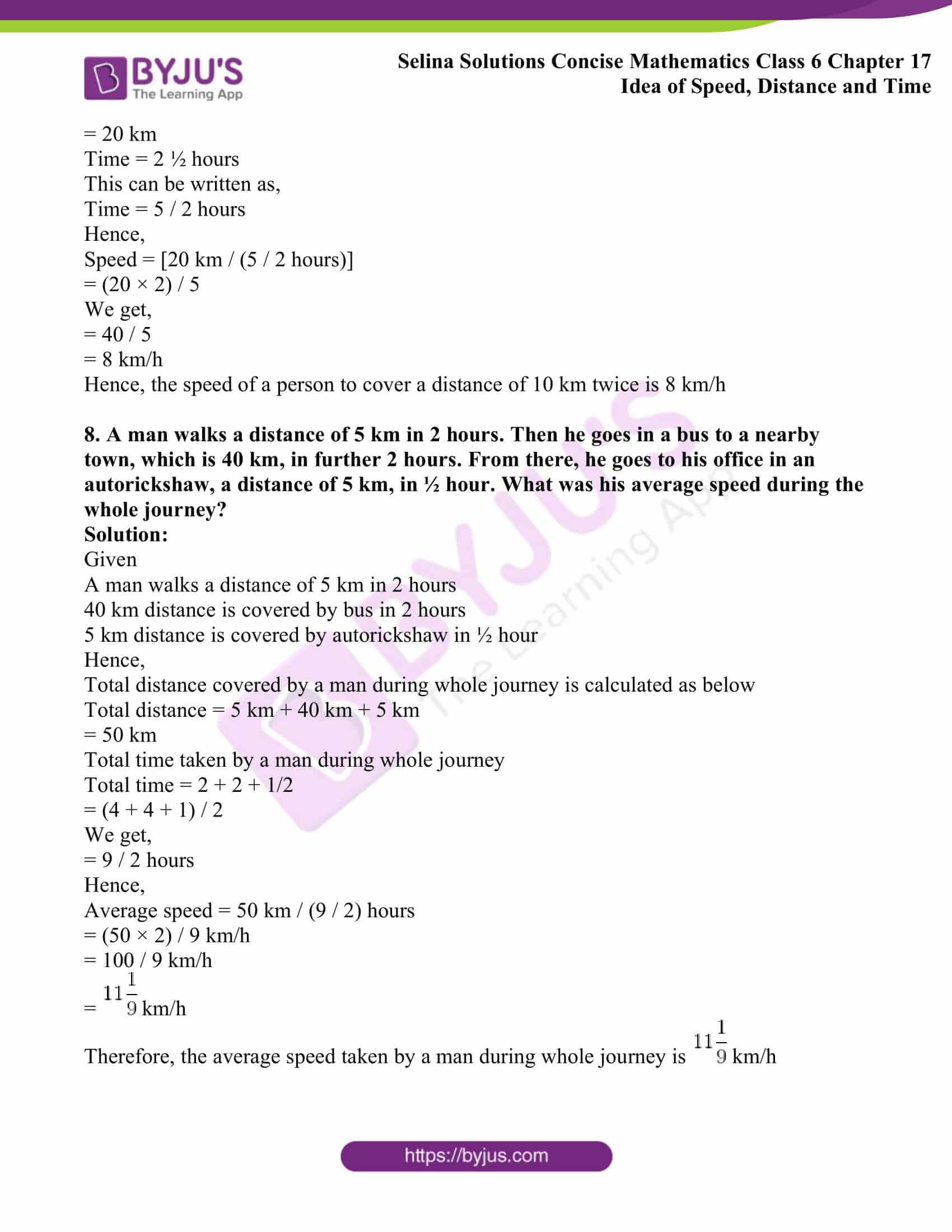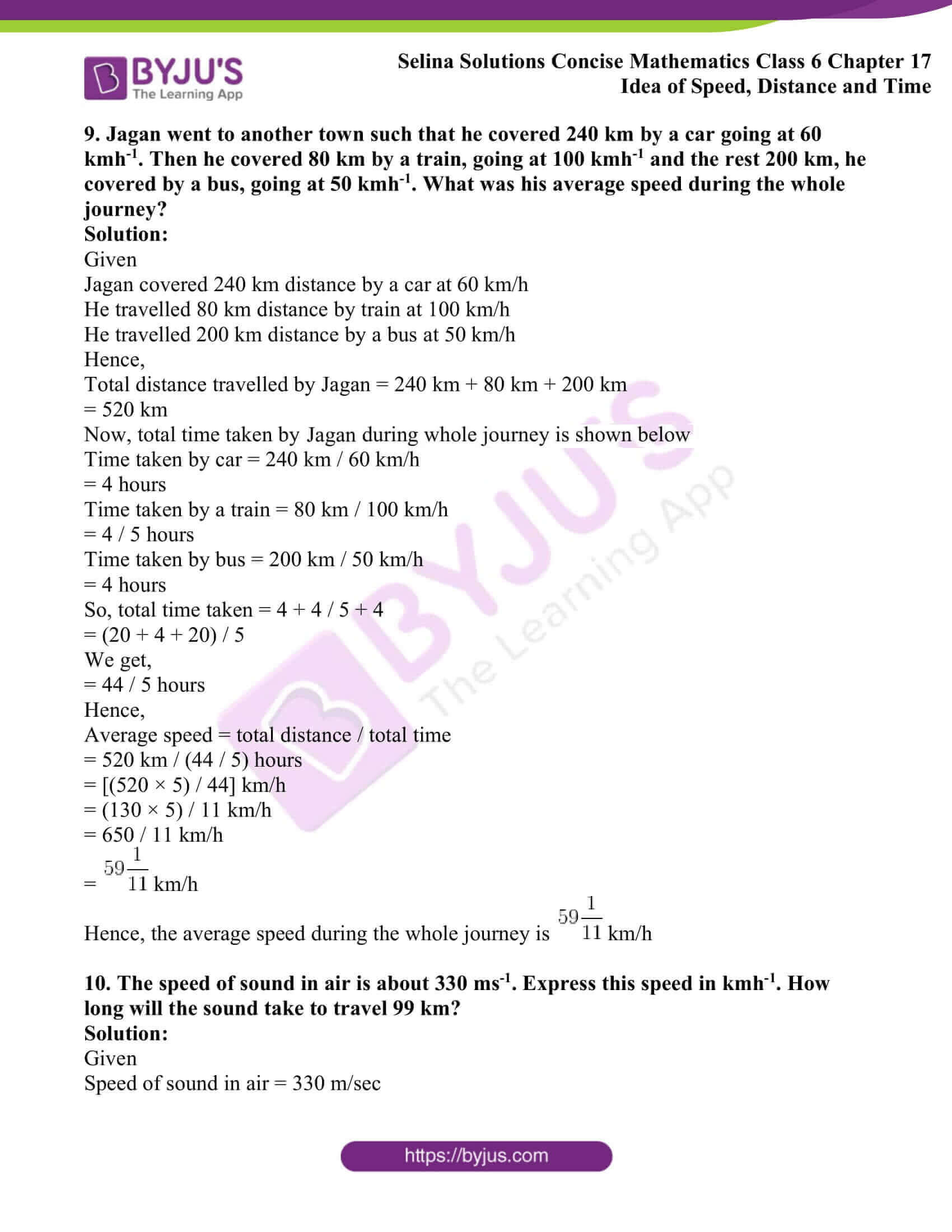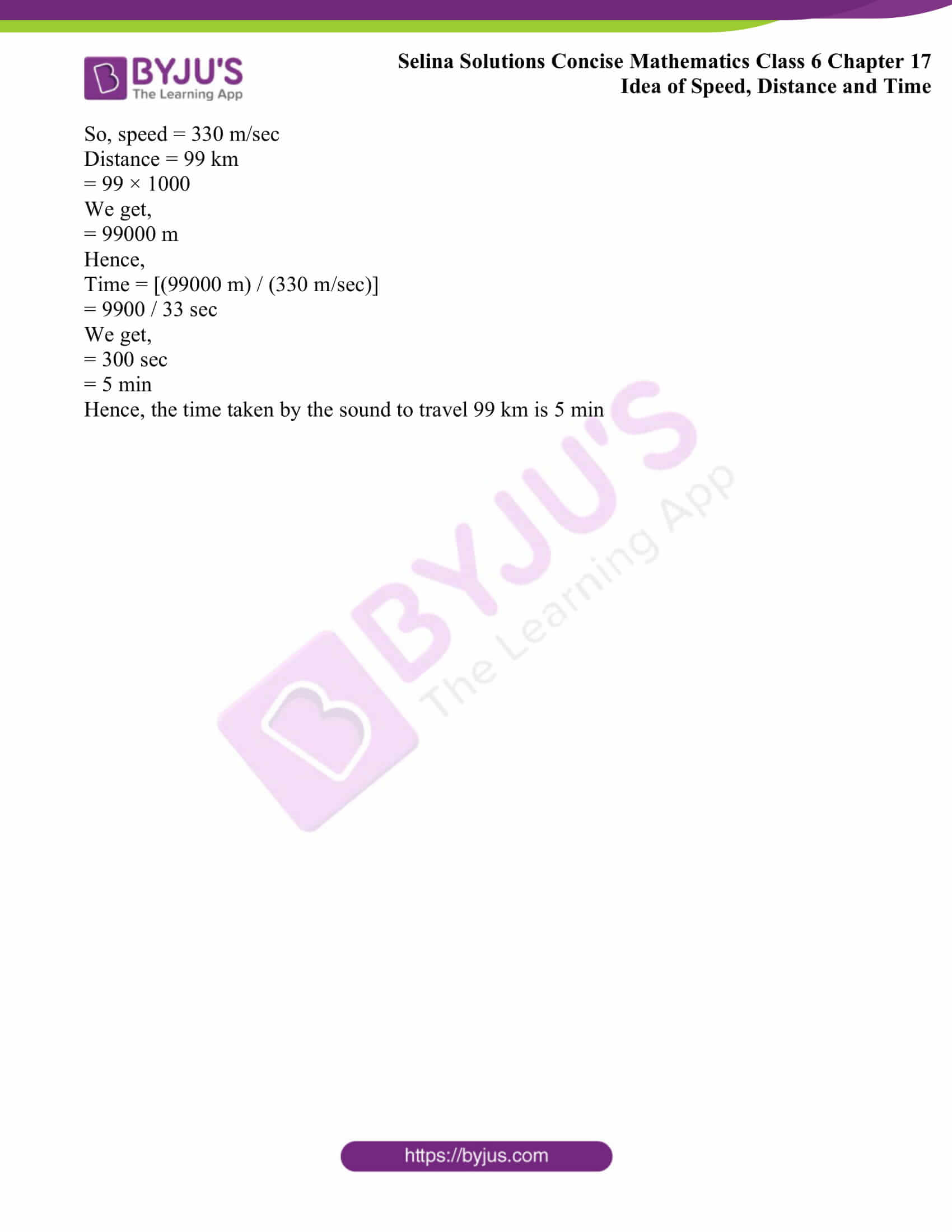### Access another exercise of Selina Solutions Concise Mathematics Class 6 Chapter 17: Idea of Speed, Distance and Time

Exercise 17(B) Solutions

### Access Selina Solutions Concise Mathematics Class 6 Chapter 17: Idea of Speed, Distance and Time Exercise 17(A)

#### Exercise 17(A)

1. A train covers 51 km in 3 hours. Calculate its speed. How far does the train go in 30 minutes?

Solution:

Given

A train covers 51 km in 3 hours

So, distance = 51 km

Time = 3 hours

Hence,

Speed = 51 km/ 3 hours

We get,

= 17 km/h

Now for 30 minutes

Speed = 17 km/h

Time = 0.5 hours

Hence,

Distance = 17 × 0.5

We get,

= 8.5 km

Therefore, train covered 8.5 km in 30 minutes

2. A motorist travelled the distance between two towns, which is 65 km, in 2 hours and 10 minutes. Find his speed in metre per minute.

Solution:

Given

A motorist travelled the distance 65 km in 2 hours and 10 minutes

So, distance = 65 km

Time = 2 hours 10 minutes

Hence,

Speed = 65 km / (2 hours + 10 minutes)

We know that,

1 km = 1000 m

1 hour = 60 min

= 65 (1000 m) / [2 (60 min) + 10 min]

= 65000 m/130 min

We get,

= 500 m/min

Therefore, the speed of a motorist in meter per minute is 500 m/min

3. A train travels 700 metres in 35 seconds. What is its speed in km/h?

Solution:

Given

A train travels 700 metres in 35 seconds

So, distance = 700 m

Time = 35 sec

Hence,

Speed = 700 m / 35 sec

1000 m = 1 km

1 m = 0.001 km

700 m = 0.001 × 700

= 0.7 km

3600 seconds = 1 hour

1 sec = 1 / 3600 hour

35 sec = 35 / 3600

Now,

Speed = Distance / Time

= [0.7 / (35/ 3600)]

We get,

= (0.7 × 3600) / 35

= 2520 / 35

= 72 km/h

Therefore, the speed of train is 72 km/h

4. A racing car covered 600 km in 3 hours 20 minutes. Find its speed in metre per second. How much distance will the car cover in 50 sec?

Solution:

Given

A racing car covered 600 km in 3 hours and 20 minutes

So, distance = 600 km

Time = 3 hours 20 minutes

Hence,

Speed = 600 km / (3 hours + 20 minutes)

= 600 (1000m) / [3(60 min) + 20 min]

We get,

= 600000m / 200 min

= 600000m / 200 (60 sec)

We get,

= 600000 / 12000 sec

= 50 m / sec

Thus, speed in meter per second is 50 m / sec

Now, the distance covered by car in 50 sec is calculated as below

Speed = 50 m / sec

Time = 50 sec

Hence,

Distance = 50 × 50

= 2500 m

= 2.5 km

Therefore, the distance covered by car in 50 seconds is 2.5 km

5. Rohit goes 350 km in 5 hours. Find:

(i) his speed

(ii) the distance covered by Rohit in 6.2 hours

(iii) the time taken by him to cover 210 km

Solution:

(i) his speed

Given

Rohit goes 350 km in 5 hours

So, distance = 350 km

Time = 5 hours

Hence,

Speed = 350 km / 5 hours

We get,

= 70 km/h

Hence, Rohit speed is 70 km/h

(ii) the distance covered by Rohit in 6.2 hours

Given

Rohit goes 350 km in 5 hours

So, distance = 350 km

Time = 5 hours

Hence,

Speed = 350 km / 5 hours

We get,

= 70 km/h

Now, the distance covered by Rohit in 6.2 hours is calculated as below

Speed = 70 km/h

Time = 6.2 hours

Hence,

Distance = 70 × 6.2

= 434 km

Therefore, Rohit covers 434 km in 6.2 hours

(iii) the time taken by him to cover 210 km

Given

Rohit goes 350 km in 5 hours

So, distance = 350 km

Time = 5 hours

Hence,

Speed = 350 km / 5 hours

We get,

= 70 km/h

Now, the time taken by Rohit to cover 210 km is calculated as below

Distance = 210 km

Speed = 70 km/h

Hence,

Time = 210 km / 70 km/h

= 3 hours

Therefore, the time taken by Rohit to cover 210 km is 3 hours

6. A boy drives his scooter with a uniform speed of 45 km/h. Find:

(i) the distance covered by him in 1 hour 20 min

(ii) the time taken by him to cover 108 km

(iii) the time taken to cover 900 m

(i) the distance covered by him in 1 hour 20 min

Given

Speed of scooter is 45 km/h

Speed = 45 km/h

Time = 1 hours 20 minutes

Hence,

Distance = 45 × (1 + 20 / 60)

= 45 × (1 + 1 / 3)

We get,

= 45 × 4 / 3

= 15 × 4

= 60

Hence, the distance covered by a boy in 1 hour 20 minutes is 60 km

(ii) the time taken by him to cover 108 km

Given

Speed of scooter is 45 km/h

So, speed = 45 km/h

Distance = 108 km

Hence,

Time = 108 km / 45 km/h

We get,

= 12 / 5 h

=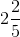h

This is equal to,

= 2 hours (2 / 5) × 60 min

= 2 hours (2 × 12) min

= 2 hours 24 min

Hence, the time taken by a boy to cover 108 km distance is 2 hours 24 min

(iii) the time taken to cover 900 m

Given

Speed of scooter is 45 km/h

So, speed = 45 km/h

Distance = 900 m

Hence,

Time = 900 m / 45 km/h

= (900 / 1000) × 1 / 45

We get,

= (9 / 10) × (1 / 45)

= 1 / 50 h

= (1 / 50) × 60 min

= 6 / 5 min

This can be written as,

= 1 and 1 / 5 min

= 1 min (1 / 5) × 60 sec

We get,

= 1 min 12 sec

Hence, the time taken by a boy to cover 900 m is 1 min 12 sec

7. I travel a distance of 10 km and come back in 2 ½ hours. What is my speed?

Solution:

Given

10 km distance travelled twice in 2 ½ hours

So, distance = 10 km + 10 km

= 20 km

Time = 2 ½ hours

This can be written as,

Time = 5 / 2 hours

Hence,

Speed = [20 km / (5 / 2 hours)]

= (20 × 2) / 5

We get,

= 40 / 5

= 8 km/h

Hence, the speed of a person to cover a distance of 10 km twice is 8 km/h

8. A man walks a distance of 5 km in 2 hours. Then he goes in a bus to a nearby town, which is 40 km, in further 2 hours. From there, he goes to his office in an autorickshaw, a distance of 5 km, in ½ hour. What was his average speed during the whole journey?

Solution:

Given

A man walks a distance of 5 km in 2 hours

40 km distance is covered by bus in 2 hours

5 km distance is covered by autorickshaw in ½ hour

Hence,

Total distance covered by a man during whole journey is calculated as below

Total distance = 5 km + 40 km + 5 km

= 50 km

Total time taken by a man during whole journey

Total time = 2 + 2 + 1/2

= (4 + 4 + 1) / 2

We get,

= 9 / 2 hours

Hence,

Average speed = 50 km / (9 / 2) hours

= (50 × 2) / 9 km/h

= 100 / 9 km/h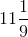km/h

Therefore, the average speed taken by a man during whole journey iskm/h

9. Jagan went to another town such that he covered 240 km by a car going at 60 kmh-1. Then he covered 80 km by a train, going at 100 kmh-1 and the rest 200 km, he covered by a bus, going at 50 kmh-1. What was his average speed during the whole journey?

Solution:

Given

Jagan covered 240 km distance by a car at 60 km/h

He travelled 80 km distance by train at 100 km/h

He travelled 200 km distance by a bus at 50 km/h

Hence,

Total distance travelled by Jagan = 240 km + 80 km + 200 km

= 520 km

Now, total time taken by Jagan during whole journey is shown below

Time taken by car = 240 km / 60 km/h

= 4 hours

Time taken by a train = 80 km / 100 km/h

= 4 / 5 hours

Time taken by bus = 200 km / 50 km/h

= 4 hours

So, total time taken = 4 + 4 / 5 + 4

= (20 + 4 + 20) / 5

We get,

= 44 / 5 hours

Hence,

Average speed = total distance / total time

= 520 km / (44 / 5) hours

= [(520 × 5) / 44] km/h

= (130 × 5) / 11 km/h

= 650 / 11 km/h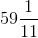km/h

Hence, the average speed during the whole journey iskm/h

10. The speed of sound in air is about 330 ms-1. Express this speed in kmh-1. How long will the sound take to travel 99 km?

Solution:

Given

Speed of sound in air = 330 m/sec

So, speed = 330 m/sec

Distance = 99 km

= 99 × 1000

We get,

= 99000 m

Hence,

Time = [(99000 m) / (330 m/sec)]

= 9900 / 33 sec

We get,

= 300 sec

= 5 min

Hence, the time taken by the sound to travel 99 km is 5 min